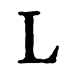This site uses cookies to deliver our services, improve performance, for analytics, and (if not signed in) for advertising. By using LibraryThing you acknowledge that you have read and understand our Terms of Service and Privacy Policy. Your use of the site and services is subject to these policies and terms.

## Related Subjects

Trigonometry (49)

Trigonometry (32)

Functions (16)

Geometry (11)

Functions (11)

Matrices (11)

Calculus (8)

Equations History (6)

## Related Tagmashes

algebra, textbook  (134)

algebra, math  (115)

math, textbook  (39)

# Subject: Algebra

### Sub-subjects

ALGEBRA (10 works)

algebra (89 works)

Algebra (203 works)

Algebra Congresses (6 works)

Algebra Congresses (249 works)

Algebra Data processing (10 works)

Algebra Data processing Congresses (1 works)

Algebra Data processing (6 works)

Algebra Early works to 1800 (5 works)

Algebra Early works to 1800 (4 works)

Algebra England History (1 works)

Algebra Graphic methods (9 works)

Algebra Graphic methods (6 works)

Algebra Great Britain History 17th century (1 works)

Algebra Great Britain History 18th century (1 works)

Algebra Handbooks, manuals, etc (1 works)

Algebra History (2 works)

Algebra History (2 works)

Algebra Juvenile literature (1 works)

Algebra Outlines, syllabi, etc (3 works)

Algebra Outlines, syllabi, etc (2 works)

Algebra Problems, exercises, etc (9 works)

Algebra Problems, exercises, etc (6 works)

Algebra Programmed instructin (1 works)

Algebra Programmed instructin (1 works)

Algebra Programmed instruction (1 works)

Algebra Programmed instruction (2 works)

Algebra Study and teaching (1 works)

Algebra Study and teaching (2 works)

Algebra Textbooks (2 works)

Algebra Textbooks (5 works)

algebra (83 works)

Algebra (101 works)

Algebra of currents (1 works)

Algebra, Abstract (72 works)

Algebra, Abstract Data processing (1 works)

Algebra, Abstract History (1 works)

Algebra, Abstract History (1 works)

Algebra, Abstract Outlines, syllabi, etc (1 works)

Algebra, Abstract Outlines, syllabi, etc (2 works)

Algebra, Abstract Textbooks (2 works)

Algebra, Abstract (21 works)

Algebra, Boolean (8 works)

Algebra, Boolean Outlines, syllabi, etc (1 works)

Algebra, Boolean (5 works)

Algebra, Homological (11 works)

Algebra, Homological Abstracts (1 works)

Algebra, Homological Congresses (1 works)

Algebra, Homological (2 works)

Algebra, Universal (7 works)

Algebra, Universal History (1 works)

Algebra, Universal History (1 works)

Algebra, Universal (1 works)

Algebraic cycles (2 works)

Algebraic fields (21 works)

Algebraic fields Congresses (1 works)

Algebraic fields (6 works)

Algebraic functions (9 works)

Algebraic functions (1 works)

Algebraic logic (2 works)

Algebraic number theory (8 works)

Algebraic number theory Congresses (1 works)

Algebraic number theory Data processing (1 works)

Algebraic number theory (1 works)

Algebraic topology (26 works)

Algebraic topology Abstracts (1 works)

Algebraic topology Congresses (2 works)

Algebraic topology History (1 works)

Algebraic topology (13 works)

Algebraic varieties (5 works)

Algebraic varieties Congresses (2 works)

Algebraic varieties (2 works)

Algebras, Linear (94 works)

Algebras, Linear Congresses (2 works)

Algebras, Linear Data processing (2 works)

Algebras, Linear Outlines, syllabi, etc (3 works)

Algebras, Linear Outlines, syllabi, etc (1 works)

Algebras, Linear Problems, exercises, etc (3 works)

Algebras, Linear Programmed instruction (2 works)

Algebras, Linear Textbooks (3 works)

Algebras, Linear Textbooks (2 works)

Algebras, Linear (50 works)

### Books under this subject

About | Contact | Privacy/Terms | Help/FAQs | Blog | Store | APIs | TinyCat | Legacy Libraries | Early Reviewers | Common Knowledge | 156,919,250 books! | Top bar: Always visible